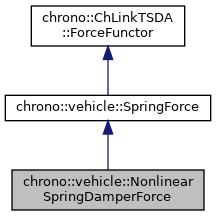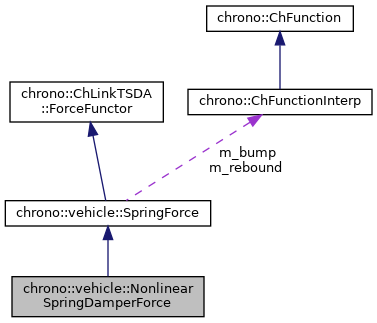chrono::vehicle::NonlinearSpringDamperForce Class Reference

## Description

Utility class for specifying a nonlinear translational spring-damper force with pre-tension.

F = P - mapK(length - rest_length) - mapC(vel)

#include <ChSubsysDefs.h>

Inheritance diagram for chrono::vehicle::NonlinearSpringDamperForce:[legend]
Collaboration diagram for chrono::vehicle::NonlinearSpringDamperForce:[legend]

## Public Member Functions

NonlinearSpringDamperForce (const std::vector< std::pair< double, double >> &dataK, const std::vector< std::pair< double, double >> &dataC, double preload=0)

void add_pointK (double x, double y)

void add_pointC (double x, double y)

virtual double evaluate (double time, double rest_length, double length, double vel, const ChLinkTSDA &link) override
Calculate and return the general spring-damper force at the specified configuration. More...Public Member Functions inherited from chrono::vehicle::SpringForce

void enable_stops (double min_length, double max_length)

void set_stops (const std::vector< std::pair< double, double >> &data_bump, const std::vector< std::pair< double, double >> &data_rebound)

void set_stops (double bump_coefficient, double rebound_coefficient)

double evaluate_stops (double length)Protected Attributes inherited from chrono::vehicle::SpringForce
double m_P
pre-tension

bool m_stops

double m_min_length

double m_max_length

ChFunction_Recorder m_bump

ChFunction_Recorder m_rebound

## ◆ evaluate()

 double chrono::vehicle::NonlinearSpringDamperForce::evaluate ( double time, double rest_length, double length, double vel, const ChLinkTSDA & link )
overridevirtual

Calculate and return the general spring-damper force at the specified configuration.

If the link has internal ODE states, the current states can be accessed with link->GetStates().

Parameters
 time current time rest_length undeformed length length current length vel current velocity (positive when extending) link associated TSDA link